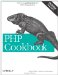# Recipe 2.14. Handling Very Large or Very Small Numbers

#### 2.14.1. Problem

You need to use numbers that are too large (or small) for PHP's built-in floating-point numbers.

#### 2.14.2. Solution

Use either the BCMath or GMP libraries.

Using BCMath:

`\$sum = bcadd('1234567812345678', '8765432187654321'); // \$sum is now the string '9999999999999999' print \$sum;`

Using GMP:

`\$sum = gmp_add('1234567812345678', '8765432187654321'); // \$sum is now a GMP resource, not a string; use gmp_strval() to convert print gmp_strval(\$sum);`

#### 2.14.3. Discussion

The BCMath library is easy to use. You pass in your numbers as strings, and the function returns the sum (or difference, product, etc.) as a string. However, the range of actions you can apply to numbers using BCMath is limited to basic arithmetic.

Another option is the GMP library. While most members of the GMP family of functions accept integers and strings as arguments, they prefer to pass numbers around as resources, which are essentially pointers to the numbers. So unlike BCMath functions, which return strings, GMP functions return only resources. You then pass the resource to any GMP function, and it acts as your number.

The only downside is when you want to view or use the resource with a non-GMP function, you need to explicitly convert it using gmp_strval( ) or gmp_intval( ).

GMP functions are liberal in what they accept. For instance, see Example 2-6.

 ``

However, you can do many more things with GMP numbers than addition, such as raising a number to a power, computing large factorials very quickly, finding a greatest common divisor (GCD), and other fancy mathematical stuff, as shown in Example 2-7.

##### Computing fancy mathematical stuff using GMP

 ``

The BCMath and GMP libraries aren't necessarily enabled with all PHP configurations. BCMath is bundled with PHP, so it's likely to be available. However, GMP isn't bundled with PHP, so you'll need to download, install it, and instruct PHP to use it during the configuration process. Check the values of function_defined('bcadd') and function_defined('gmp_init') to see if you can use BCMath and GMP. If you're using Windows, you need to be running PHP 5.1 or higher to use GMP.

Another options for high-precision mathematics is PECL's big_int library, shown in Example 2-8.

 ``

Documentation on BCMath at http://www.php.net/bc, big_int at http://pecl.php.net/big_int, and GMP at http://www.php.net/gmp.PHP Cookbook: Solutions and Examples for PHP Programmers
ISBN: 0596101015
EAN: 2147483647
Year: 2006
Pages: 445